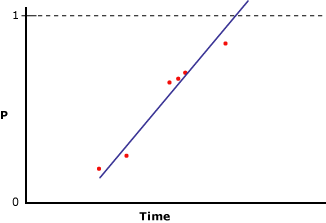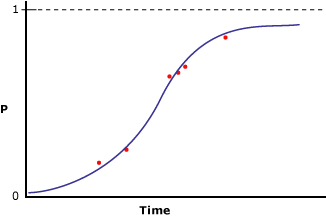# Microsoft Logistic Regression Algorithm

The Microsoft Logistic Regression algorithm is a variation of the Microsoft Neural Network algorithm, where the HIDDEN_NODE_RATIO parameter is set to 0. This setting will create a neural network model that does not contain a hidden layer, and that therefore is equivalent to logistic regression.

Suppose the predictable column contains only two states, yet you still want to perform a regression analysis, relating input columns to the probability that the predictable column will contain a specific state. The following diagram illustrates the results you will obtain if you assign 1 and 0 to the states of the predictable column, calculate the probability that the column will contain a specific state, and perform a linear regression against an input variable.The x-axis contains values of an input column. The y-axis contains the probabilities that the predictable column will be one state or the other. The problem with this is that the linear regression does not constrain the column to be between 0 and 1, even though those are the maximum and minimum values of the column. A way to solve this problem is to perform logistic regression. Instead of creating a straight line, logistic regression analysis creates an "S" shaped curve that contains maximum and minimum constraints. For example, the following diagram illustrates the results you will achieve if you perform a logistic regression against the same data as used for the previous example.Notice how the curve never goes above 1 or below 0. You can use logistic regression to describe which input columns are important in determining the state of the predictable column.

## Using the Algorithm

Use the Microsoft Neural Network Viewer to explore a linear regression mining model.

A logistic regression model must contain a key column, one or more input columns, and one or more predictable columns.

The Microsoft Logistic Regression algorithm supports specific input column content types, predictable column content types, and modeling flags, which are listed in the following table.

 Input column content types Continuous, Cyclical, Discrete, Discretized, Key, Table, and Ordered Predictable column content types Continuous, Cyclical, Discrete, Discretized, and Ordered Modeling flags MODEL_EXISTENCE_ONLY and NOT NULL

All Microsoft algorithms support a common set of functions. However, the Microsoft Logistic Regression algorithm supports additional functions, listed in the following table.

For a list of the functions that are common to all Microsoft algorithms, see Data Mining Algorithms. For more information about how to use these functions, see Data Mining Extensions (DMX) Function Reference.

Models that use the Microsoft Logistic Regression algorithm do not support drillthrough or data mining dimensions, because the structure of nodes in the mining model does not necessarily correspond directly to the underlying data.

The Microsoft Logistic Regression algorithm supports several parameters that affect the performance and accuracy of the resulting mining model. The following table describes each parameter.

Parameter Description

HOLDOUT_PERCENTAGE

Specifies the percentage of cases within the training data used to calculate the holdout error. HOLDOUT_PERCENTAGE is used as part of the stopping criteria while training the mining model.

The default is 30.

HOLDOUT_SEED

Specifies a number to use to seed the pseudo-random generator when randomly determining the holdout data. If HOLDOUT_SEED is set to 0, the algorithm generates the seed based on the name of the mining model, to guarantee that the model content remains the same during reprocessing.

The default is 0.

MAXIMUM_INPUT_ATTRIBUTES

Defines the number of input attributes that the algorithm can handle before it invokes feature selection. Set this value to 0 to turn off feature selection.

The default is 255.

MAXIMUM_OUTPUT_ATTRIBUTES

Defines the number of output attributes that the algorithm can handle before it invokes feature selection. Set this value to 0 to turn off feature selection.

The default is 255.

MAXIMUM_STATES

Specifies the maximum number of attribute states that the algorithm supports. If the number of states that an attribute has is larger than the maximum number of states, the algorithm uses the most popular states of the attribute and ignores the remaining states.

The default is 100.

SAMPLE_SIZE

Specifies the number of cases to be used to train the model. The algorithm provider uses either this number or the percentage of total of cases that are not included in the holdout percentage as specified by the HOLDOUT_PERCENTAGE parameter, whichever value is smaller.

In other words, if HOLDOUT_PERCENTAGE is set to 30, the algorithm will use either the value of this parameter, or a value that is equal to 70 percent of the total number of cases, whichever is smaller.

The default is 10000.